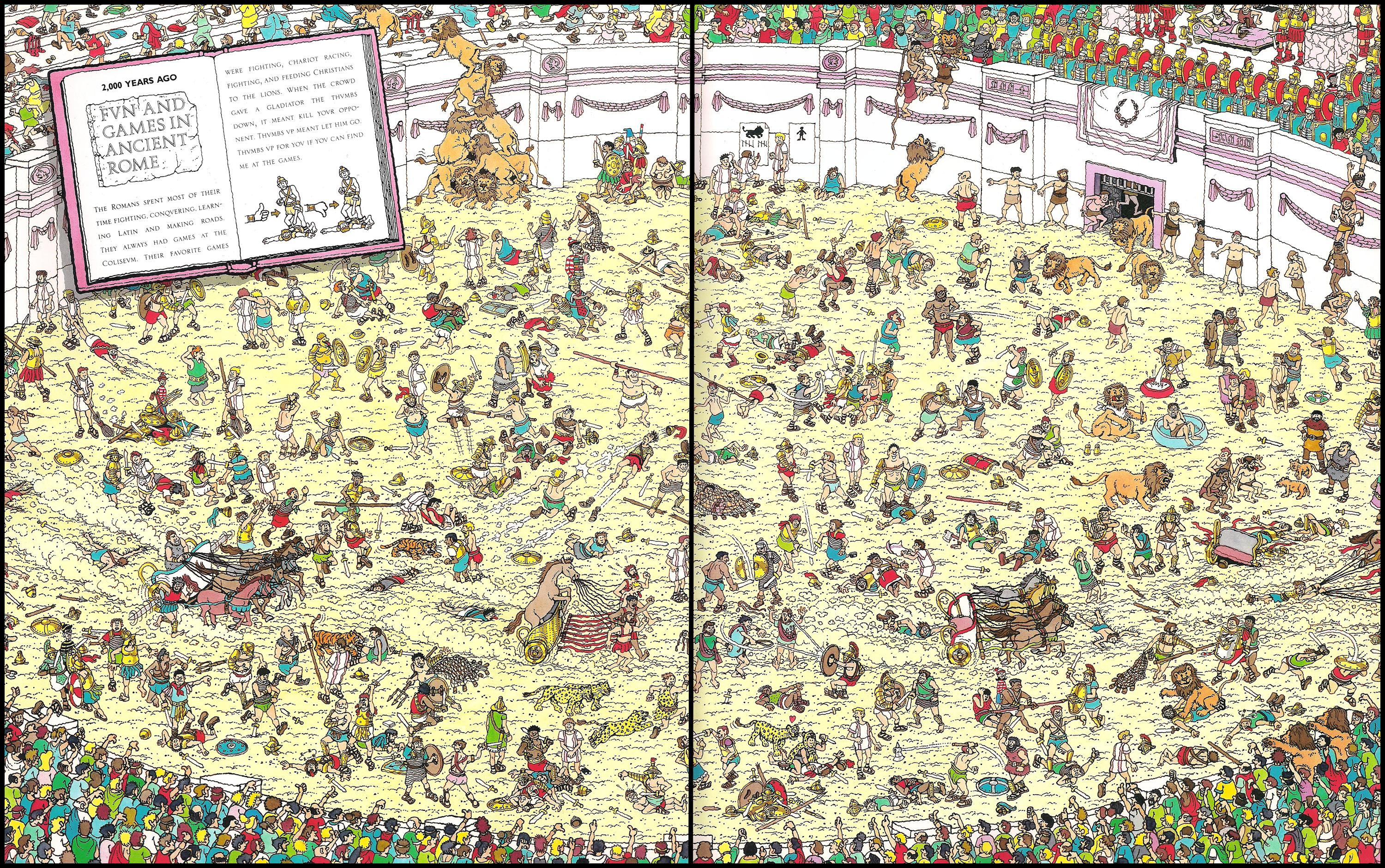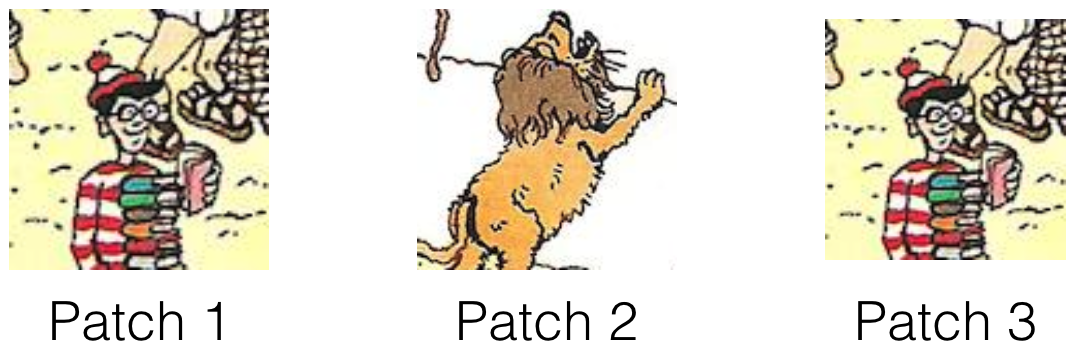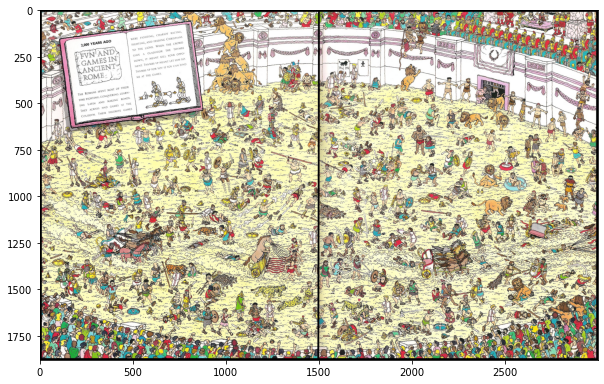# Template Matching¶

Faisal Qureshi
Professor
Faculty of Science
Ontario Tech University
http://vclab.science.ontariotechu.ca

## Lesson Plan¶

• Sum of squared differences
• Normalized sum of squared differences
• Cross-correlation
• Normalized cross-correlation
• Correlation coefficient
• Normalized correlation coefficient

## Where is waldo?¶

Our task is to find Waldoin the crowd### Key insight¶

Compare patches in the image with Waldo's picture. In order to do so, we need to be able determine if patch 1 is more similar to patch 2 or patch 3 (as shown in the figure below).### Template Matching¶

Given a source image $I$ and a template $T$, we compare the template image against the source image by sliding it one pixel at a time (left to right, top to bottom) and computing a similarity (or alternately difference) between template and the image patch at each location. The similarity scores is computed using a suitable function $g(T, I, i, j) \mapsto R(i,j)$, where $R(i,j)$ is the similarity score for between the template and the image patch at location $(i,j)$. Location corresponding to highest (or alternately lowest) value in the result matrix $R$ represent the "match location."

A common trick is to treat both the template and patches as vectors in a high-dimensional space. Template-patch-matching problem is then reduced to finding the nearest vector (in this high-dimensional space).

## Picking image patches¶

In :
import cv2
import numpy as np
import scipy as sp
from scipy import signal
import matplotlib.pyplot as plt

In :
import numpy as np
import cv2 as cv
import matplotlib.pyplot as plt

I = cv.cvtColor(I, cv.COLOR_BGR2RGB)
plt.figure(figsize=(10,10))
plt.imshow(I)

Out:
<matplotlib.image.AxesImage at 0x13b291210>Lets pick a patch from this image

In :
y, x = 250, 750
half_h, half_w = 100, 200

def pick_patch(I, y, x, half_h, half_w):
return I[y-half_h:y+half_h+1, x-half_w:x+half_w+1, :]

for yi in range(3):
for xi in range(1):
x_ = x + 30 * xi
y_ = y + 30 * yi
patch = pick_patch(I, y_, x_, half_h, half_w)
fig = plt.figure(figsize=(5,5))
plt.imshow(patch)
plt.title('Patch: yx = {}, size = {}'.format((y_,x_), patch.shape[:2]))
plt.show()